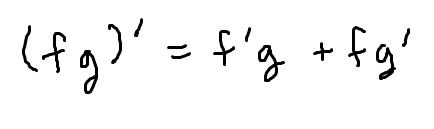# The product rule for derivatives

Much of calculus and finding derivatives is about determining which rule applies to which case. The product rule, simply put, is applied when your function is the product of two other functions.In this guide, we will look at how to remember the product rule, how to recognize when it should be used, and finally, how to use it.

## Remembering the product rule

There is an easy trick to remembering this important rule: write the product out twice (adding the two terms), and then find the derivative of the first term in the first product and the derivative of the second term in the second product.## Examples

The easiest way to understand when this applies and how to use it is to look at some examples. In each case, pay special attention to how we identify that we are looking at a product of two functions.

### Example

Find the derivative of the function.

$$f(x) = x^4\ln(x)$$

### Solution

This function is the product of two simpler functions: $$x^4$$ and $$\ln(x)$$. Therefore, we can apply the product rule to find its derivative.

Write the product out twice, and put a prime on the first and a prime on the second:

$$\left(f(x)\right)^{\prime} = \left(x^4\right)^{\prime}\ln(x) + x^4\left(\ln(x)\right)^{\prime}$$

Take the derivatives using the rule for each function. Remember that for $$x^4$$, you will apply the power rule and that the derivative of $$\ln(x)$$ is $$\dfrac{1}{x}$$.

\begin{align}\left(f(x)\right)^{\prime} &= \left(x^4\right)^{\prime}\ln(x) + x^4\left(\ln(x)\right)^{\prime}\\ &= \left(4x^3\right)\ln(x) + x^4\left(\dfrac{1}{x}\right)\end{align}

Simplify, if possible.

\begin{align}&= \left(4x^3\right)\ln(x) + x^3\\ &= \boxed{x^3\left(4\ln(x) + 1\right)}\end{align}

Either of the last two lines can be used as a final answer, but the last one looks a little nicer and is probably going to be preferred by your teacher if you are currently taking calculus!

Let’s look at another example to make sure you got the basics down.

### Example

Find the derivative of the function.

$$y = 2xe^x$$

This is the product of $$2x$$ and $$e^x$$, so we apply the product rule. Remember that the derivative of $$2x$$ is 2 and the derivative of $$e^x$$ is $$e^x$$.

\begin{align}y^{\prime} &= \left(2x\right)^{\prime}e^x + 2x\left(e^x\right)^{\prime}\\ & = 2e^x + 2xe^x\\ &= \boxed{2e^x\left(1 + x\right)}\end{align}

As you can see, with product rule problems, you are really just changing the derivative question into two simpler questions.

## Hint: Watch for shortcuts

This hint could also be called “now that you know the product rule, don’t go blindly applying it”. To understand what that means, consider the following function:

$$y = \left(x+4\right)\left(x+1\right)$$

This is a product of $$x+4$$ and $$x+1$$, so if we want to find the derivative, we should use the product rule, right?

It’s true – you could use that. However, it is more work than recognizing that, by FOILing you get:

$$y = \left(x+4\right)\left(x+1\right) = x^2+5x + 4$$

Then by applying the power rule you have:

$$y^\prime = \left(x^2+5x + 4\right)^{\prime} = 2x + 5$$

This is the kind of thing you want to learn to notice. There are many problems where you can save yourself some calculus workby simplifying ahead of time. In the examples before, however, that wasn’t possible, and so the product rule was the best approach.